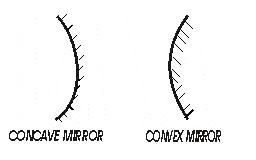# Physics for IITJEE:Optics Short Notes-1

Agenda :Summary of the basics of Optics

Reflection of light
-When light travelling in one medium is incident on a second medium some part of it is sent back to the first medium according to some definite laws and is said to be reflected.
-In practice mirrors are used to reflect light.
-The angle which the incident ray and the reflected ray make with normal to the surface are termed as Angles of incidence and of reflection respectively.

Laws of reflection:-
Law 1- The incident ray, the reflected ray and the normal to the surface , all lie in the same plane.
Law 2- The angle of incidence i is equal to the angle of reflection r.

Spherical Mirrors :-
Assuming that all the reflecting surfaces are portions of a sphere, if the inside surface reflects, the mirror is concave and if the outside surface which reflects, it is convex as shown below in the figure.
-Relation between focal length f and radius of curvature R of the mirror is
f=R/2
and both f and R are taken as positive for concave mirror and negative for convex mirror.
-Mirror formula is the relation between distance of the object (u), distance of the image (v) and the focal length (f) of the mirror and is,
(1/u)+(1/v)=(1/f)
-Mirror formula holds for a spherical mirror of small aperture only.
-The ratio of size of the image formed (by spherical mirror) to the size of the object is called magnification. Mathematically,
m=I/O
=-v/u
-Spherical abberation is the inability of a spherical mirror of large aperture to bring all the rays of wide beam of light falling

on it to focus at a single point.